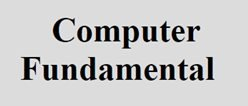# EBCDIC Code and ASCII Code

Here you will learn about EBCDIC Code and ASCII Code one by one.

## EBCDIC Code

Extended Binary Coded Decimal Interchange (EBCDIC) code is 8 bits code which means 28 = 256 ways of representing digits, alphabets and special symbol.

Parity bit is also used under the system. EBCDIC is an 8 bit code, it can be divided into two 4 bit group. Each of these 4 bit group represented by hexadecimal number system is used as sort out notation for memory dump by computer, which is used EBCDIC for internal representation of character. This result in a one to four year reduction in the volume of memory dump.

## ASCII Code

American Standard Code for Information Interchange (ASCII) code is ways of representing digits, alphabets and special symbols.

In the ASCII code, the leftmost bit is used as parity bit (cheek bit) and is not used to characters. Each digits in a numeric value is stored in a single byte. This code is popular in data communication, is used almost exclusively to represent data internally in microprocessor, and is frequently found in the larger computer produced by some vendor.

### Types of ASCII Code

ASCII is of two types:

1. ASCII-7
2. ASCII-8

In a ASCII-7 code, first three bit are known as zone bits and rest bits represent digits value. A latest version of ASCII is known as ASCII-8, that is it is a 8 bit code, 256 character can be coded.

In ASCII-8, the first four bits are known as zone bits and rest four bits represent digit values.

Tools
Calculator